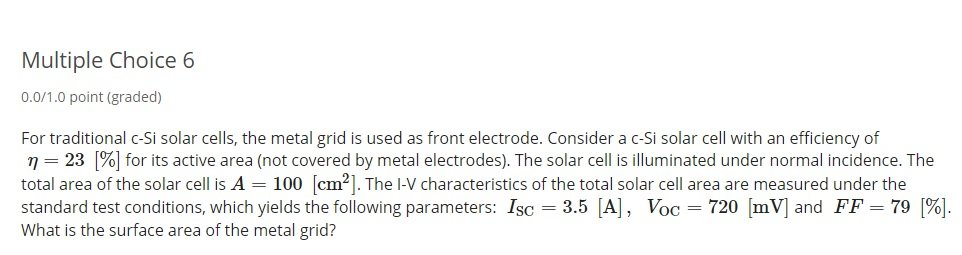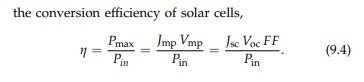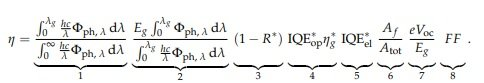## Recommended Posts

Hello all,

I'm looking for insight with one of my solar engineering course problems, the answers are multiple choice. I'll post the problems and the relevant (unless I'm missing some pieces) equations below, as well as explain the logic of my thought process. This is a multiple choice problem, and I already solved for one of the available choices however I more or less am looking for some validation in the process... if I had to guess I'm missing something still.

Here the assumption is that the incident power (P_in) under standard testing conditions is 1000W/m^2, and that J_sc = I_sc / area.

From there I used the equation in the second picture and basically can plug and chug, to solve for the MAX efficiency of the entire solar cell, provided I am given the maximum area. I'll denote the efficiency as n_max.

n_max = (I_sc/area)(V_oc)(FF)/P_in

n_max = (3.5 A / 0.01 m^2)(0.72V)(79%) / (1000 W/m^2)

n_max = 0.199 = 19.9%

It makes sense to me that this n_max value is smaller than the n provided of the total active surface area of 23%, since efficiency will be lost due to the metal grid. From the third picture below bracket 6 is the quantity associated with losses due to metal electrode covering, shadowing. I.E I used only that part of the equation, in order to solve for A_f which essentially what I was hoping to be the answer.

n_max = (n_active area) (A_f/A_total) =

0.199 = (0.23) (A_f/100cm^2) ; A_f = 86.55cm^2

Unfortunately, or perhaps fortunately, this isn't one of the choices available.

However, 0.199 = (A_f/100cm^2) ; A_f = 19.9 cm^2 DOES happen to be an option. It just doesn't make sense to me, the given information the active surface efficiency being 23% doesn't help me and would be filler information.

Another thought process of mine is to solve for A_f by using the efficiency of 23%, and instead of an area of 100cm^2 I would use (100 - A_f) cm^2 and solve for area.

If there are any suggestions to how to solve for A_f it would be greatly appreciated.

Best,

LestI solved this problem going a different route, thank you anyway. I won't delete just in case someone is encouraged for the challenge!

Best,

Lest

## Create an account

Register a new account